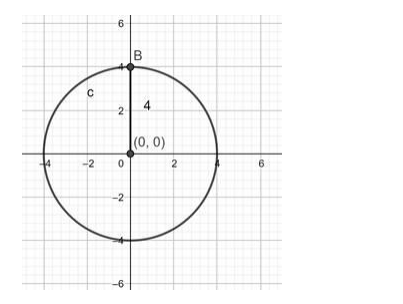# Find the equation of a circle withQuestion:

Find the equation of a circle with

Centre at the origin and radius 4

Solution:

The general form of the equation of a circle is:

$(x-h)^{2}+(y-k)^{2}=r^{2}$

Where, (h, k) is the centre of the circle.

r is the radius of the circle.

Substituting the centre and radius of the circle in he general form:

$\Rightarrow(x-0)^{2}+(y-0)^{2}=4^{2}$

$\Rightarrow x^{2}+y^{2}=16$Ans; equation of a circle with. Centre at the origin and radius 4 is:

$x^{2}+y^{2}=16$